Scroll

# Basic analysis of MaxDiff data

Follow
 Summary This article contains an example MaxDiff data file. There is a portfolio included which can be used to carry out a basic analysis using the 'paired preference' calculation. Applies to askiaanalyse Written for Data processors, researchers, statisticians. Keywords maxdiff; analyse; paired preference; conditional formatting; edits routing; t-test; 95% confidence level; significant difference; least; most; significance; best-worst scaling; transposed table.

This relates to the article in the Design section of the our help centre on how to script a MaxDiff example.

First we will show the additions required to that .qex file to eventually transform the collected data into a form which is easier to analyse: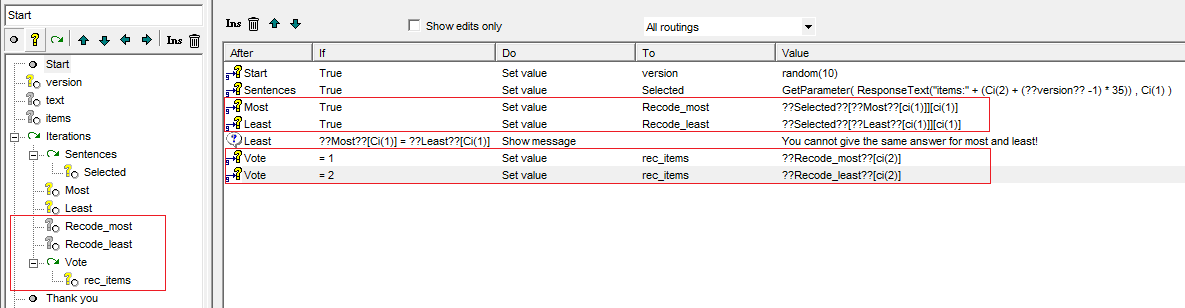Everything boxed in red is a new addition to the original file. First we edit the data for the most/least into a full list of attributes/statements:

Most/Least: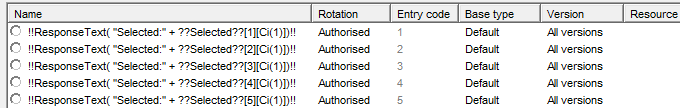Recode_most/Recode_least: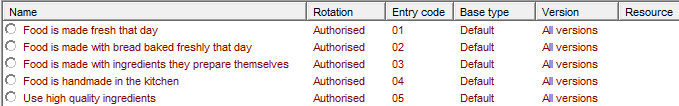Then we edit the data for the two variables Recode_most/Recode_least into a loop where the codes, most and least, are in the loop question level (Vote) and the 35 attributes/statements are in the child question level (rec_items). This allows us to run a summary table of the loop with more flexibility on which statistical tests we can use.

Now set-up a transposed table as below - this transposition makes the % down effectively appear as % across. Why do we do this?

We are going to use the Paired preference calculation which is basically a col sig test between the most and least columns and returns the t-test value between them. This calculation must be based on % down. However, it's more useful for us to see % across saying least/most.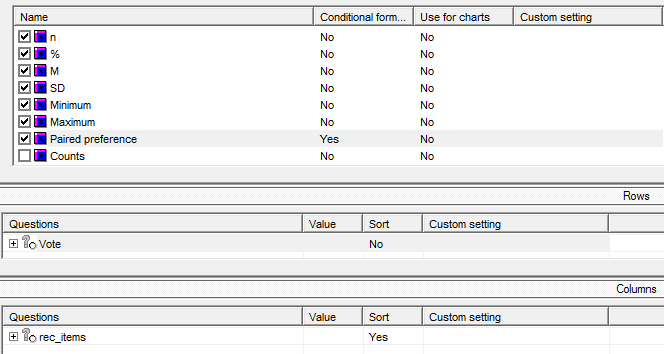Since the t-test value is returned, it's useful to use some conditional formatting to show when the values are < -1.96 or > 1.96 i.e. significant at the 95% confidence level. That's done by right-clicking on the calculation > Conditional formatting and inserting your rules: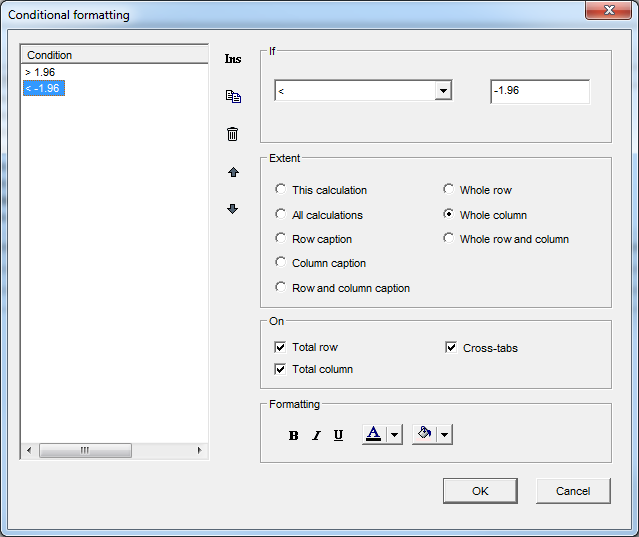The result when you run your table:The biggest difference between most favoured and least favoured attribute/statement is represented by a green row and a higher paired preference value. These would be the attributes to concentrate on improving in the grocery store example above. The least important are the red rows with a lower paired preference value.

The example .qes file and portfolio can be found in the attached MaxDiff_Example_Analysis.zip